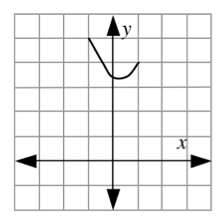### Home > APCALC > Chapter 7 > Lesson 7.4.3 > Problem7-204

7-204.

$y=f(x)$ satisfies $\frac{dy}{dx}=2x$ and its graph contains the point $(-1,5)$.

1. Use Euler’s Method to sketch an approximation of $y=f(x)$ over $-1\le x\le1$. Use $Δx=0.5$.

Show all steps and carefully record the coordinate points at $x=−0.5$, $x=0$, $x=0.5$, and $x=1$.2. Use implicit integration to solve the differential equation.

Use $(−1,5)$ to help you find the value of $C$ after you integrate.

3. Compare your answers to parts (a) and (b). Determine the largest difference between the actual $y$-value and the Euler’s Method $y$-value for $x=-1$, $-0.5$, $0$, $0.5$, and $1$. This largest difference estimates the error of Euler’s Method. If $Δx=0.1$ instead, do you think this error will be larger or smaller?